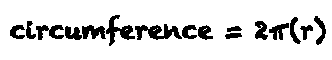# Circle Circumference Calculator

## Circle Circumference

#### For Circles with Known Radius

Result:
31.416decimals

A circle with a radius of 5 units has a circumference of 31.416 units.

This calculator and more easy to use calculators waiting at www.KylesCalculators.com### Calculating the Circumference of a Circle:

The circumference of a circle with a known radius and can calculated using the following formula:

C = 2Π(r)

The constant PI (Π) can be approximated as 3.14159, but your calculator probably has a button for this.

See the example below to calculate the circumference of a circle with a radius of 5. If your calculator doesn't have a PI (Π) function you can substitute 3.14159.

• C = 2pi(r)
• = 2pi(5)
• = 2(15.7079633)
• = 31.4159266
• ~ 31.42

### Radius and Calculating from Diameter

Radius is the measured distance from the centre of a circle to the edge. If you don't know the radius but you do know the total width of the circle finding the radius is a snap. The circle's width is known as diameter. Radius can easily be calculated from diameter by dividing it in half because diameter is passing through the centre of the circle from edge to edge it is twice as large as radius (it is made up of two radii).

Here is an example of calculating radius given a circle with a diameter of 8.

• r = d / 2
• = 8 / 2
• = 4